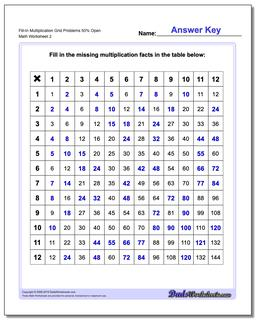# Math Worksheets: Multiplication: Multiplication: Fill-In Multiplication Grid Problems 50% Open (Second Worksheet)## Fill-In Multiplication Grid Problems 50% Open (Second Worksheet)

PropertyValue
DescriptionFill-In Multiplication Grid Problems 50% Open: Grid problem multiplication worksheets for introducing multiplication and discovering patterns in multiplication problems (Answer keys to these sheets make a great multiplication fact chart!) (Second Worksheet)
Resource TypeWorksheet